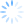How does our Microsoft Excel add-in, CDXZipStream, determine the straight-line distance between two points?  This question applies to the following right-click functions:

CDXDistance - calculates the distance between two zip codes

CDXDistance2WP - calculates the distance between two points of latitude and longitude, also referred to as waypoints

CDXClosestZip – determines which zip code out of a list is closest to a target location

(For calculating driving distance, please refer to our function CDXRouteMP, shown in the Youtube tutorial Driving Distance Calculator in Excel. )

Technically, it’s not a straight line distance in these cases, since we need to take into account the fact that the earth is (approximately) spherical in shape.  To do this, both CDXDistance and CDXDistance2WP use a mathematical equation for the shortest distance along a spherical surface, called the Great Circle formula:

d = a cos-1[cosA1 CosA2 cos(B1 – B2) + sin A1 SinA2]

Where

d = the shortest path between two points on a sphere, also called the orthodrome

a = the radius of the earth, which is 3958.73926185 miles

A1, A2 = the latitude of points 1 and 2

B1, B2 = the longitude of points 1 and 2

For the functions CDXDistance and CDXClosestZip, which both require zip codes as input, the centroid location of each zip code area is used as the basis for the latitude and longitude points.

Note that this is an approximation of the distance, since the earth is not a perfect sphere (it's actually a lumpy ellipsoid), and changes in altitude along the earth’s surface will also impact the actual distance.  When the latitude and longitude are provided in decimal format, this equation can be converted into the following Excel-friendly formula: